# Comprehensive Guide to Scatter Plot using ggplot2 in R

• Last Updated : 23 Feb, 2022

In this article, we are going to see how to use scatter plots using ggplot2 in the R programming language.

ggplot2 package is a free, open-source, and easy-to-use visualization package widely used in R. It is the most powerful visualization package written by Hadley Wickham. This package can be installed using the R function install.packages().

`install.packages("ggplot2")`

A scatter plot uses dots to represent values for two different numeric variables and is used to observe relationships between those variables. To plot scatterplot we will use we will be using geom_point() function. Following is brief information about ggplot function, geom_point().

Syntax : geom_point(size, color, fill, shape, stroke)

Parameter :

• size : Size of Points
• color : Color of Points/Border
• fill : Color of Points
• shape : Shape of Points in in range from 0 to 25
• stroke : Thickness of point border
• Return : It creates scatterplots.

Example: Simple scatterplot

## R

 `library``(ggplot2)` `ggplot``(iris, ``aes``(x = Sepal.Length, y = Sepal.Width)) +` `    ``geom_point``()`

Output:## Scatter plot with groups

Here we will use distinguish the values by a group of data (i.e. factor level data). aes() function controls the color of the group and it should be factor variable.

Syntax:

aes(color = factor(variable))

Example: Scatterplot with groups

## R

 `# Scatter plot with groups`   `ggplot``(iris, ``aes``(x = Sepal.Length, y = Sepal.Width)) +` `    ``geom_point``(``aes``(color = ``factor``(Sepal.Width)))`

Output: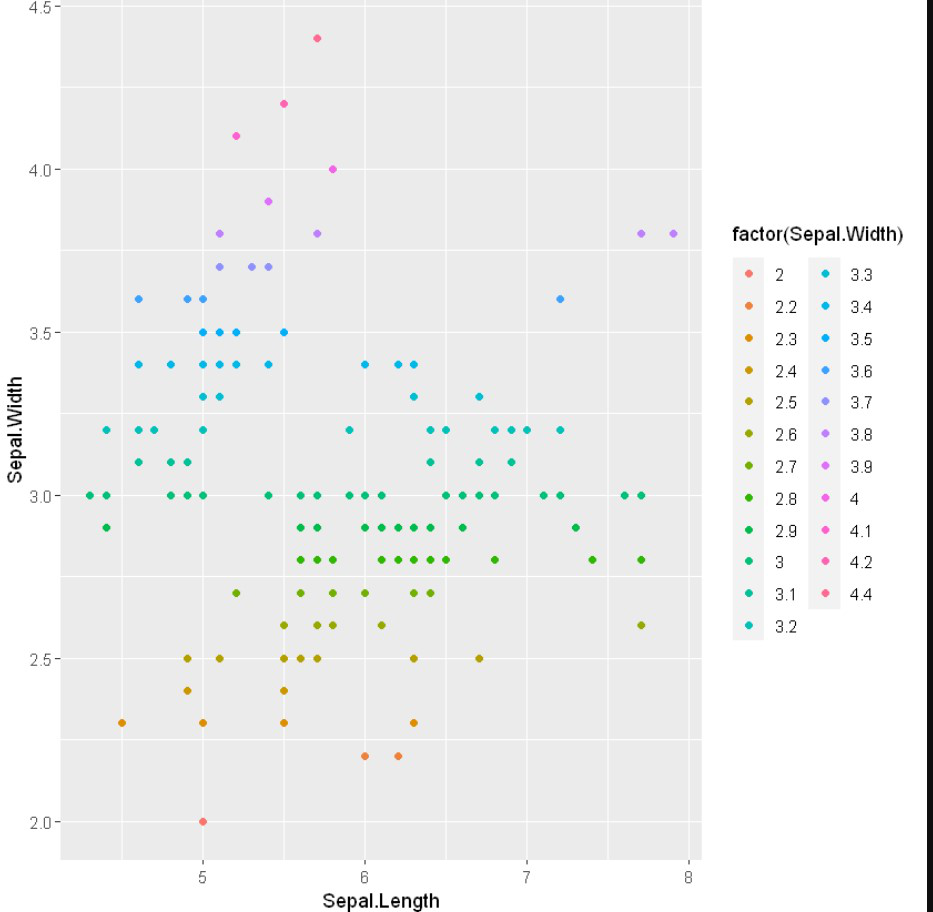## Changing color

Here we use aes() methods color attributes to change the color of the datapoints with specific variables.

Example: Changing color

## R

 `# Changing color`   `ggplot``(iris) +` `    ``geom_point``(``aes``(x = Sepal.Length, ` `                   ``y = Sepal.Width, ` `                   ``color = Species))`

Output: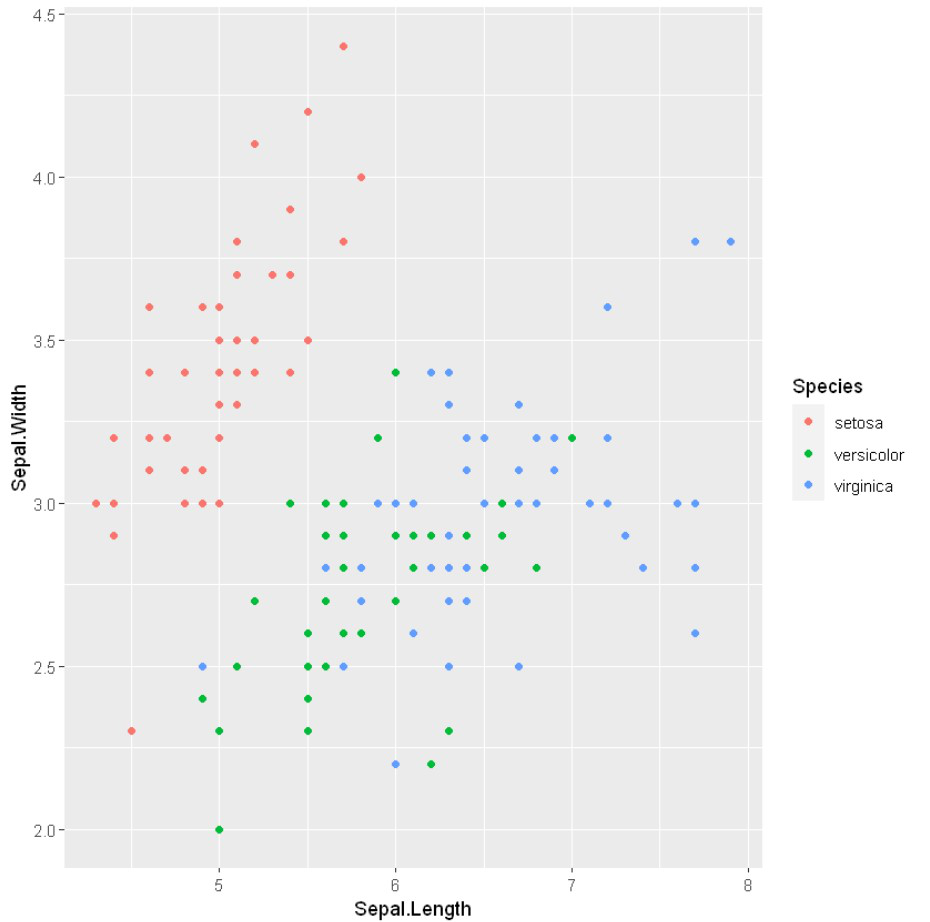## Changing Shape

To change the shape of the datapoints we will use shape attributes with aes() methods.

Example: Changing shape

## R

 `# Changing point shapes in a ggplot scatter plot` `# Changing color`   `ggplot``(iris) +` `    ``geom_point``(``aes``(x = Sepal.Length, y = Sepal.Width, ` `                   ``shape = Species , color = Species))`

Output: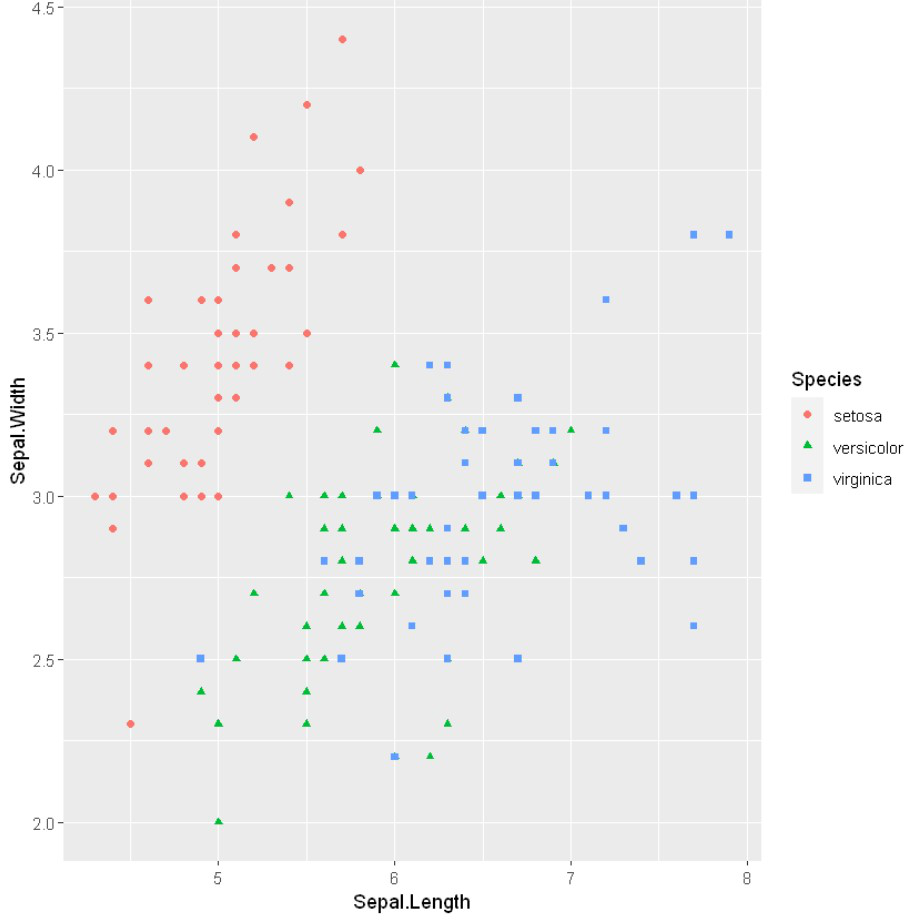## Changing the size aesthetic

To change the aesthetic or datapoints we will use size attributes in aes() methods.

Example: Changing size

## R

 `# Changing the size aesthetic mapping in a ` `# ggplot scatter plot`   `ggplot``(iris) +` `    ``geom_point``(``aes``(x = Sepal.Length,` `                   ``y = Sepal.Width,` `                   ``size = .5))`

Output: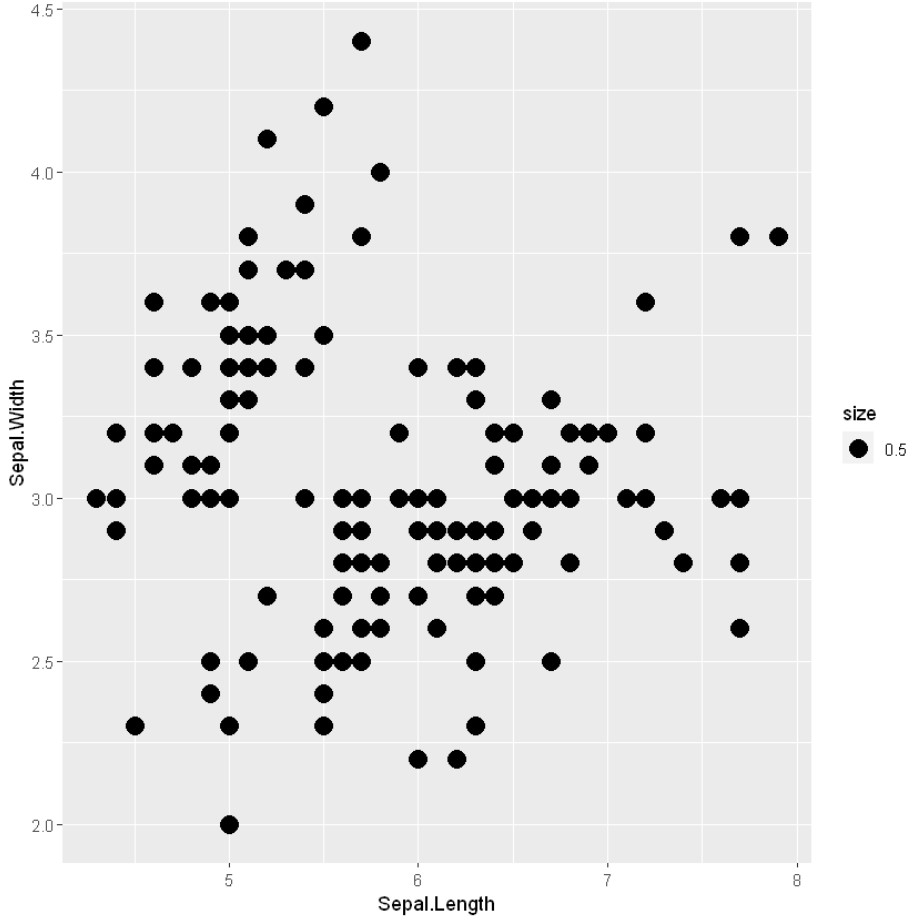## Label points in the scatter plot

To deploy the labels on the datapoint we will use label into the geom_text() methods.

Example: Label points in the scatter plot

## R

 `# Label points in the scatter plot`   `ggplot``(iris, ``aes``(x = Sepal.Length, y = Sepal.Width)) +` `    ``geom_point``() +` `    ``geom_text``(label=``rownames``(iris))`

Output: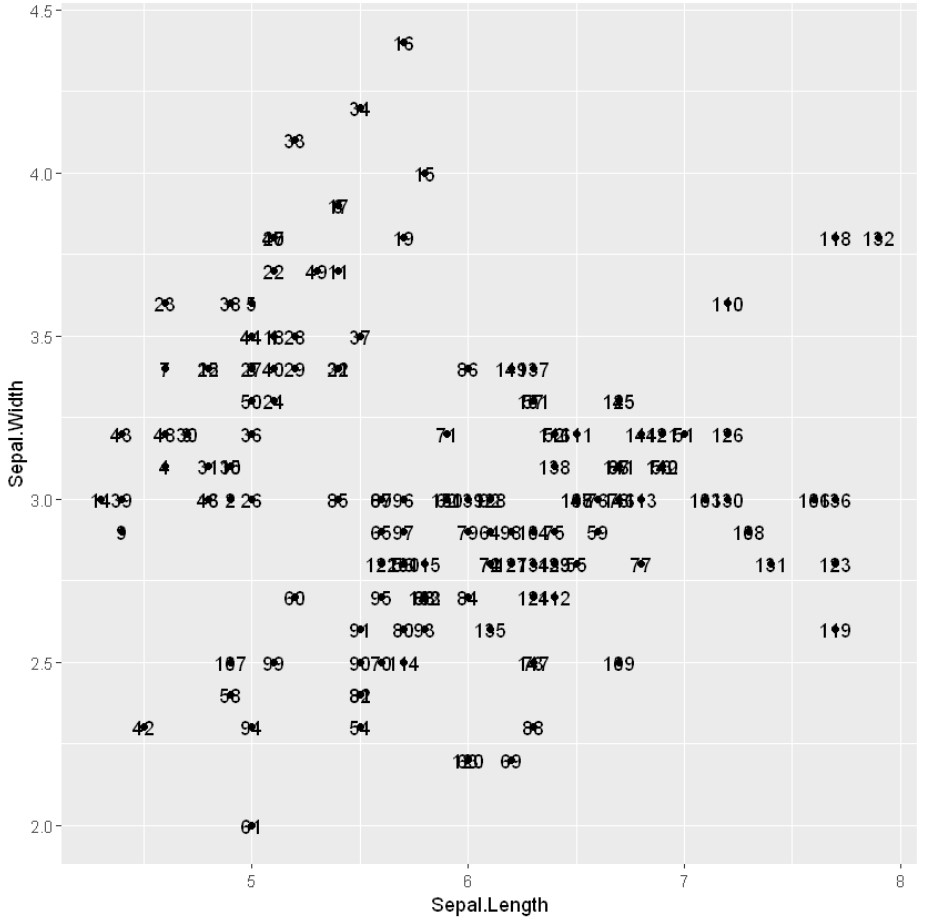## Regression lines in ggplot2

Regression models a target prediction value supported independent variables and mostly used for finding out the relationship between variables and forecasting. In R we can use the stat_smooth() function to smoothen the visualization.

Syntax: stat_smooth(method=”method_name”, formula=fromula_to_be_used, geom=’method name’)

Parameters:

• method: It is the smoothing method (function) to use for smoothing the line
• formula: It is the formula to use in the smoothing function
• geom: It is the geometric object to use display the data

Example: Regression line

## R

 `# Add regression lines with stat_smooth` `ggplot``(iris, ``aes``(x = Sepal.Length, y = Sepal.Width)) +` `    ``geom_point``() + ` `    ``stat_smooth``(method=lm)`

Output: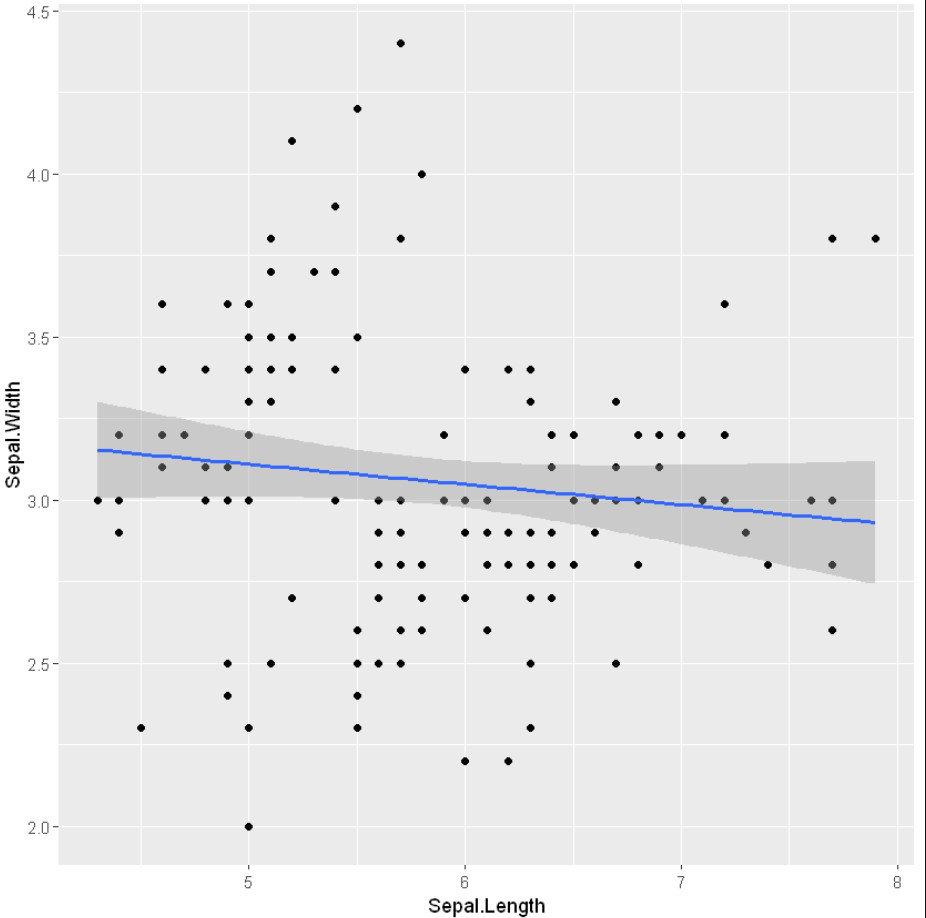Example: Using stat_mooth with loess mode

## R

 `# Add regression lines with stat_smooth` `ggplot``(iris, ``aes``(x = Sepal.Length, y = Sepal.Width)) +` `    ``geom_point``() + ` `    ``stat_smooth``()`

Output: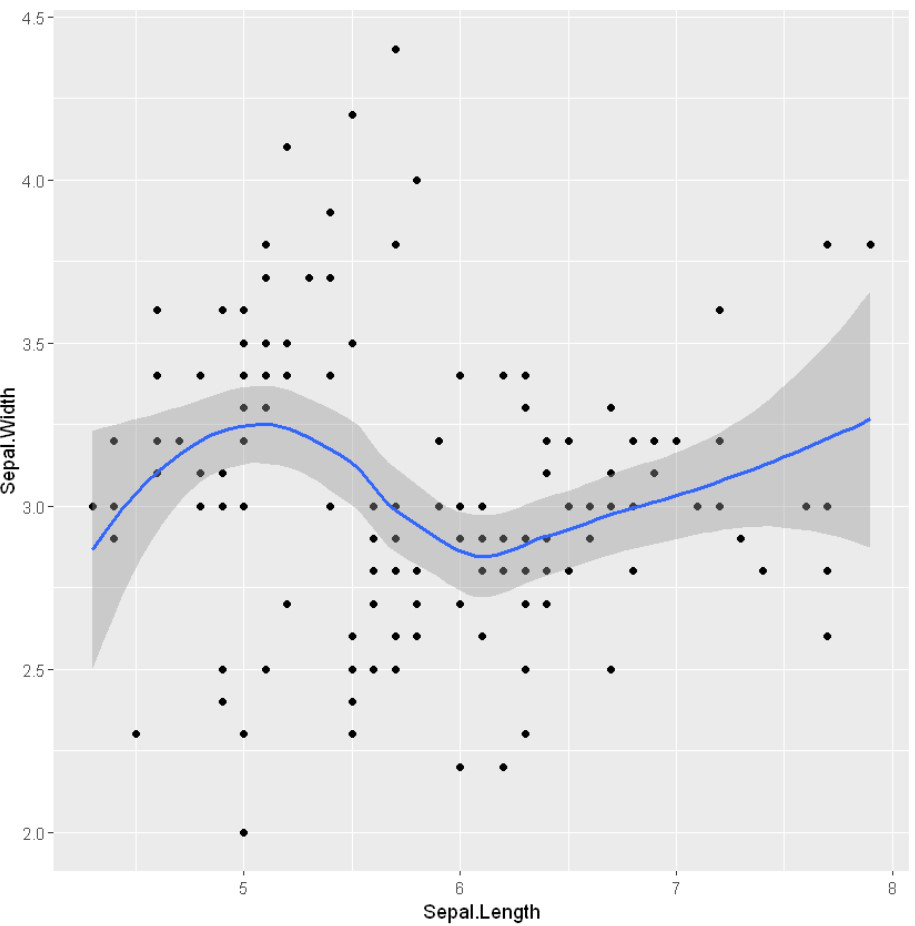geom_smooth() function to represent a regression line and smoothen the visualization.

Syntax: geom_smooth(method=”method_name”, formula=fromula_to_be_used)

Parameters:

• method: It is the smoothing method (function) to use for smoothing the line
• formula: It is the formula to use in the smoothing function

Example: Using geom_smooth()

## R

 `# Add regression lines with geom_smooth` `ggplot``(iris, ``aes``(x = Sepal.Length, y = Sepal.Width)) +` `    ``geom_point``() + ` `    ``geom_smooth``()`

Output:In order to show the regression line on the graphical medium with help of geom_smooth() function, we pass the method as “loess” and the formula used as y ~ x.

Example: geom_smooth with loess mode

## R

 `# Add regression lines with geom_smooth` `ggplot``(iris, ``aes``(x = Sepal.Length, y = Sepal.Width)) + ` `    ``geom_point``() + ` `    ``geom_smooth``(method=lm, se=``FALSE``)`

Output: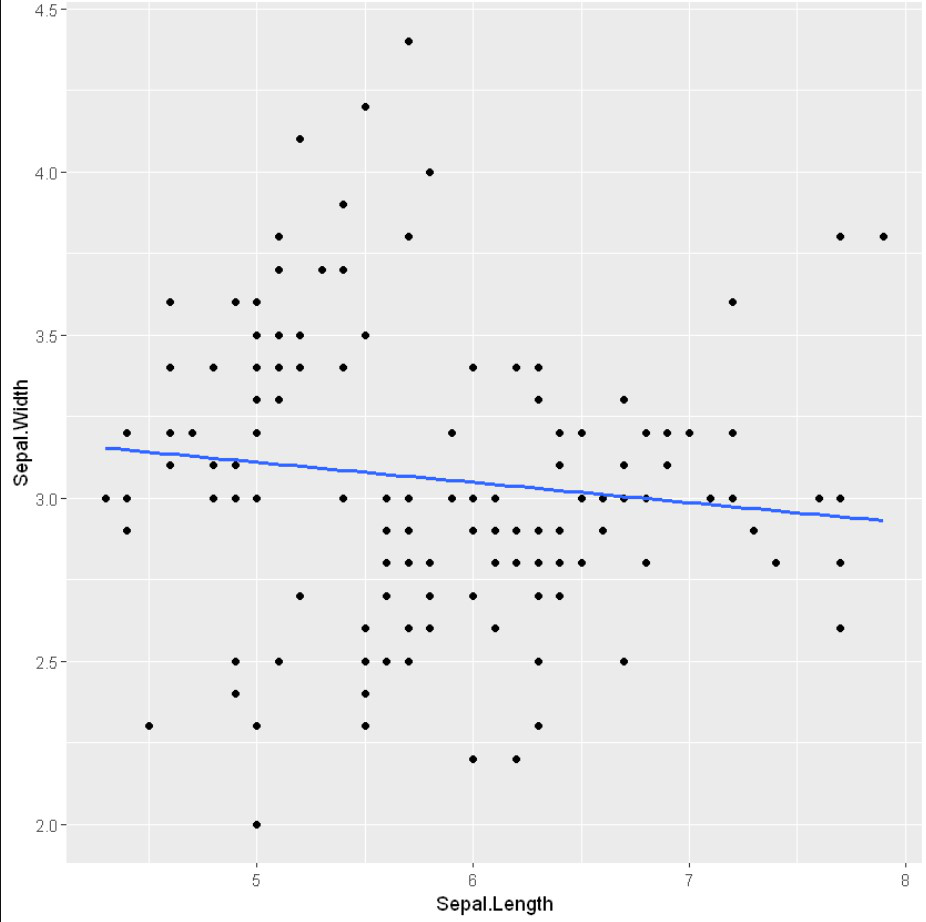The intercept and slope can be easily calculated by the lm() function which is used for linear regression followed by coefficients().

Example: Intercept and slope

## R

 `# Add regression lines with geom_smooth` `ggplot``(iris, ``aes``(x = Sepal.Length, y = Sepal.Width)) +` `    ``geom_point``() + ` `    ``geom_smooth``(intercept = 37, slope = -5, color=``"red"``, ` `                 ``linetype=``"dashed"``, size=1.5)`

Output: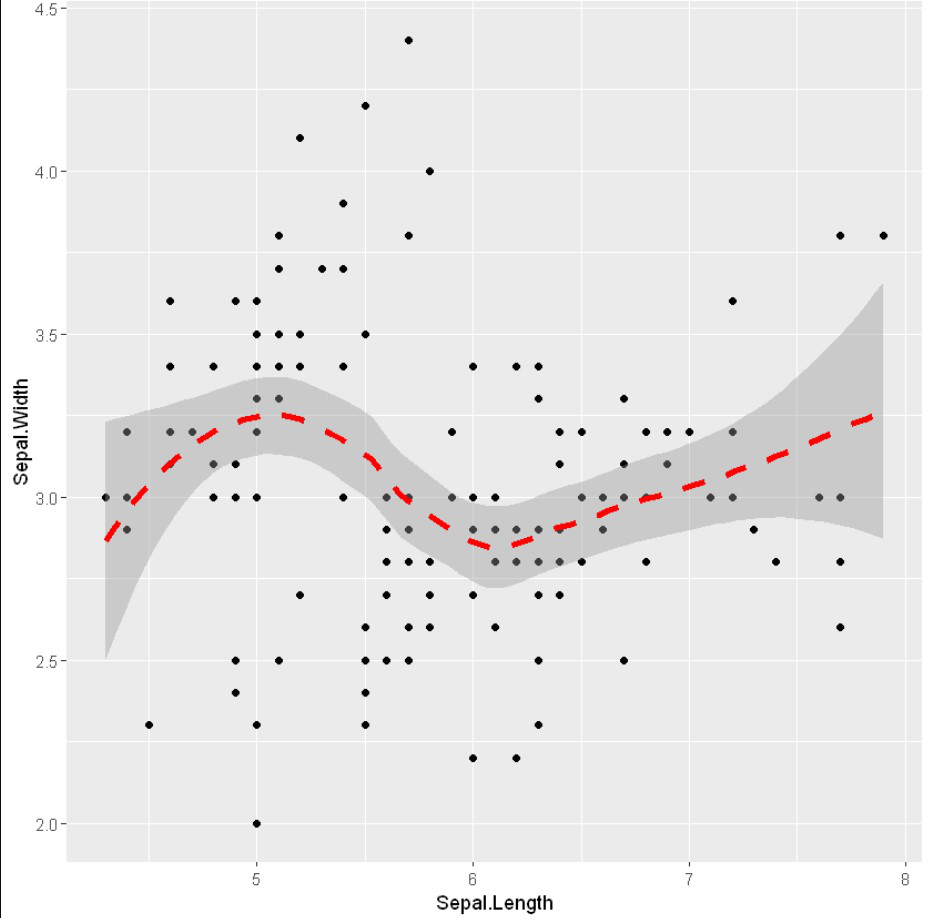## Change the point color/shape/size manually

scale_fill_manual, scale_size_manual, scale_shape_manual, scale_linetype_manual, are builtin types which is assign desired colors to categorical data, we use one of them scale_color_manual() function, which is used to scale (map).

Syntax :

• scale_shape_manualValue) for point shapes
• scale_color_manual(Value) for point colors
• scale_size_manual(Value) for point sizes

Parameter :

• values : A set of aesthetic values to map the data. Here we take desired set of colors.

Return : Scale the manual values of colors on data

Example: Changing aesthetics

## R

 `# Change the point color/shape/size manually` `library``(ggplot2)`   `# Change point shapes and colors manually` `ggplot``(iris, ``aes``(x = Sepal.Length, y = Sepal.Width, color = Species)) +` `    ``geom_point``() +` `    ``geom_smooth``(method=lm, se=``FALSE``, fullrange=``TRUE``)+` `    ``scale_shape_manual``(values=``c``(3, 16, 17))+ ` `    ``scale_color_manual``(values=``c``(``'#999999'``,``'#E69F00'``, ``'#56B4E9'``))+` `    ``theme``(legend.position=``"top"``)`

Output: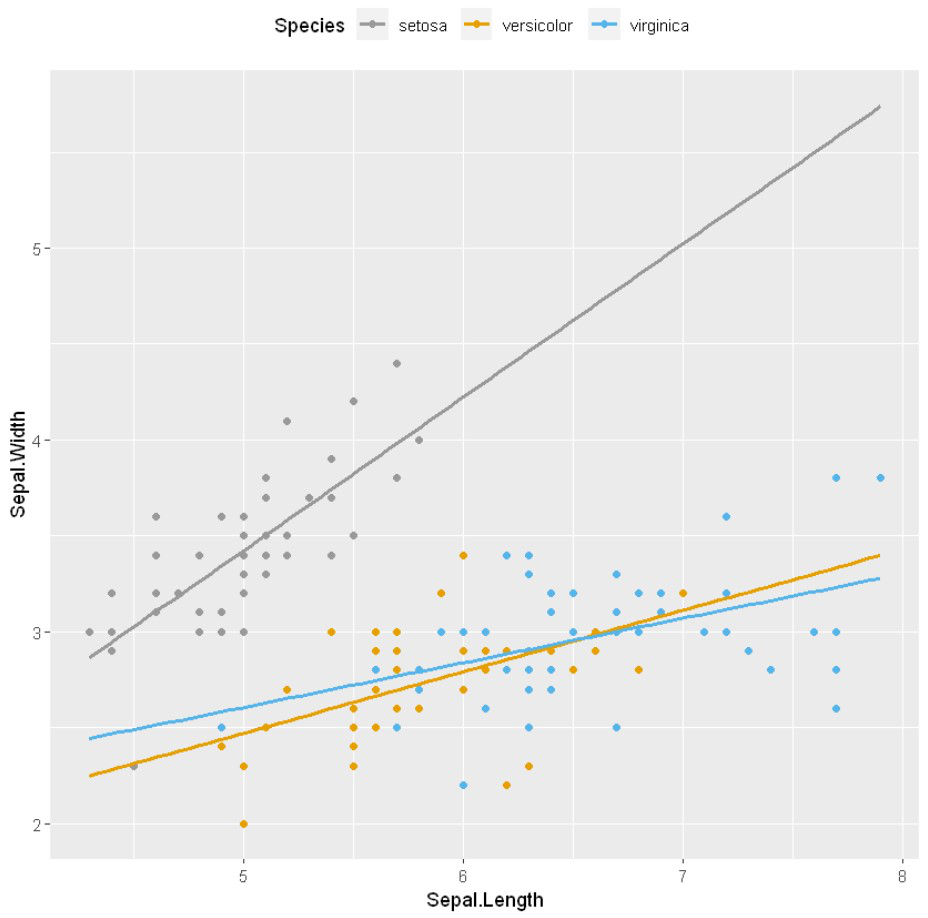## Marginal rugs to a scatter plot

To add marginal rugs to the scatter plot we will use geom_rug() methods.

Example: Marginal rugs

## R

 `# Add marginal rugs to a scatter plot` `# Changing point shapes in a ggplot scatter plot` `# Changing color`   `ggplot``(iris) +` `    ``geom_point``(``aes``(x = Sepal.Length, y = Sepal.Width,` `                   ``shape = Species , color = Species))+` `    ``geom_rug``()`

Output: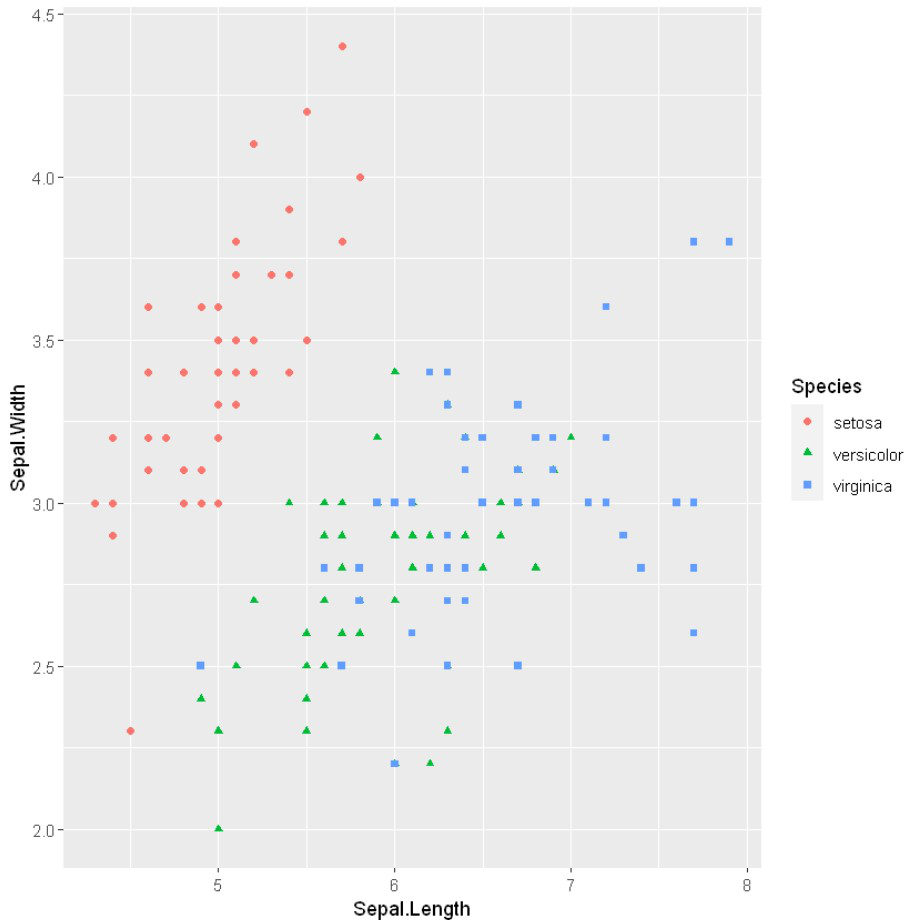Here we will add marginal rugs into the scatter plot

Example: Marginal rugs

## R

 `# Add marginal rugs to a scatter plot`   `ggplot``(iris, ``aes``(x = Sepal.Length, y = Sepal.Width)) +` `    ``geom_point``()+` `    ``geom_rug``()`

Output: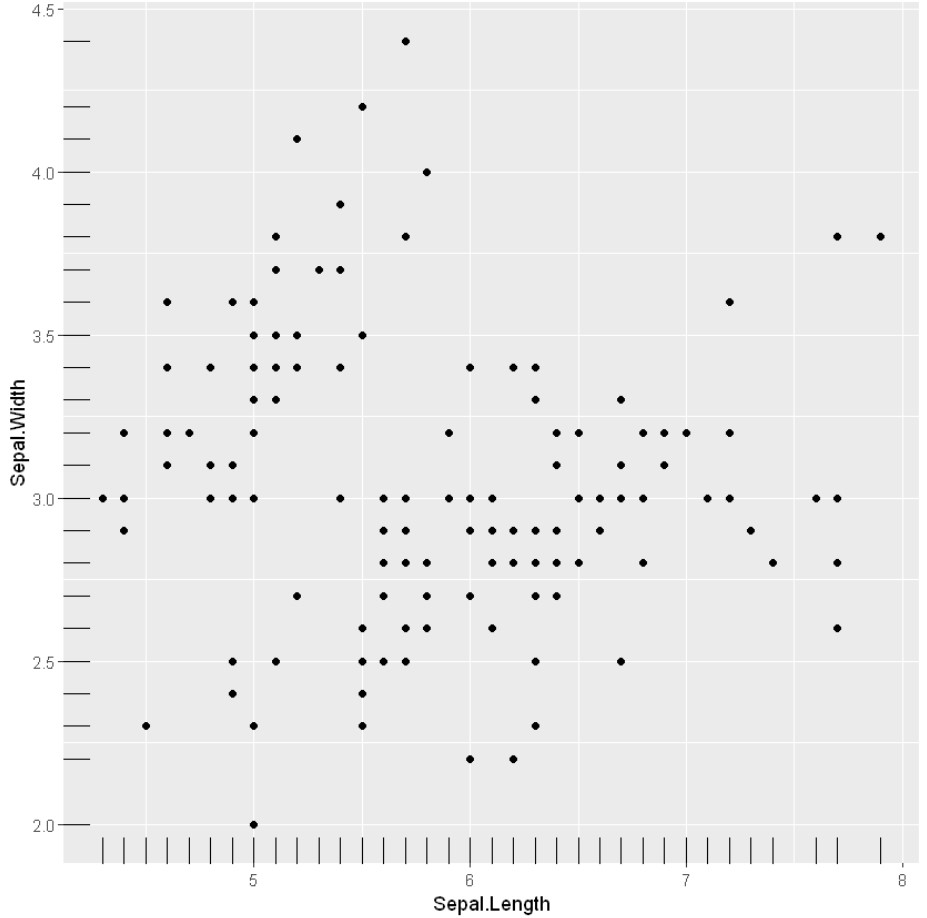## Scatter plots with the 2-D density estimation

To create density estimation in scatter plot we will use geom_density_2d() methods and geom_density_2d_filled() from ggplot2.

Syntax: ggplot( aes(x)) + geom_density_2d( fill, color, alpha)

Parameters:

• fill: background color below the plot
• color: the color of the plotline
• alpha: transparency of graph

Example: Scatterplots with 2-D density estimation

## R

 `# Scatter plots with the 2d density estimation`   `ggplot``(iris, ``aes``(x = Sepal.Length, y = Sepal.Width)) +` `    ``geom_point``()+` `    ``geom_density_2d``()`

Output: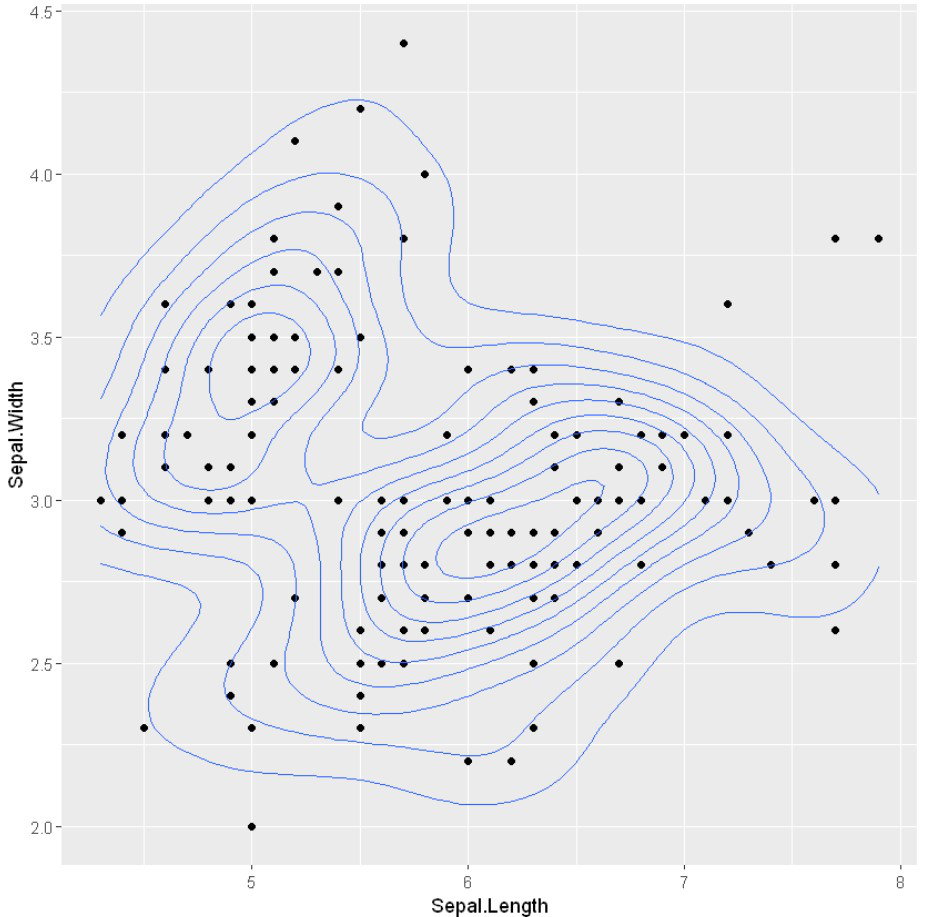Using geom_density_2d_filled() to visualize the situation of color inside the datapoints

## R

 `ggplot``(iris, ``aes``(x = Sepal.Length, y = Sepal.Width)) +` `    ``geom_point``()+` `    ``geom_density_2d``(alpha = 0.5)+` `    ``geom_density_2d_filled``()`

Output: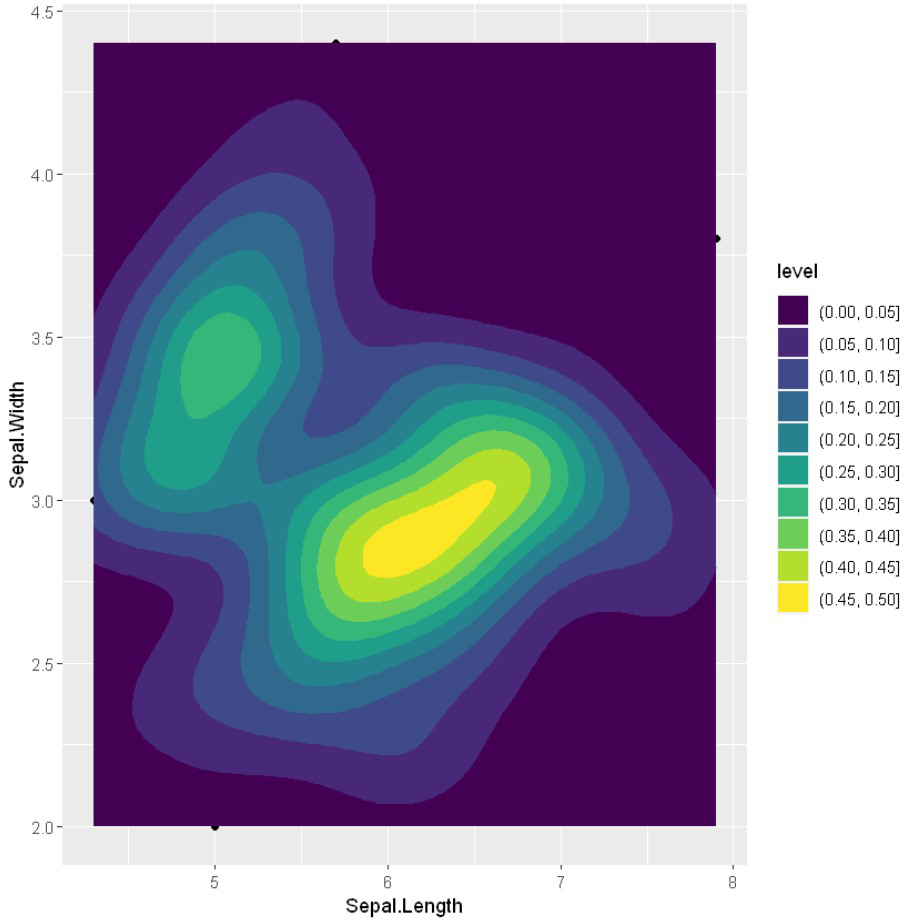stat_density_2d() can be also used to deploy the 2d density estimation.

Example: Deploy density estimation

## R

 `# Scatter plots with the 2d density estimation`   `ggplot``(iris, ``aes``(x = Sepal.Length, y = Sepal.Width)) +` `    ``geom_point``()+` `    ``stat_density_2d``()`

Output:## Scatter plots with ellipses

To add a circle or ellipse around a cluster of data points, we use the stat_ellipse() function. This function automatically computes the circle/ellipse radius to draw around the cluster of points by categorical data.

Example: Scatterplot with ellipses

## R

 `# Scatter plots with ellipses`   `ggplot``(iris, ``aes``(x = Sepal.Length, y = Sepal.Width)) +` `    ``geom_point``()+` `    ``stat_ellipse``()`

Output: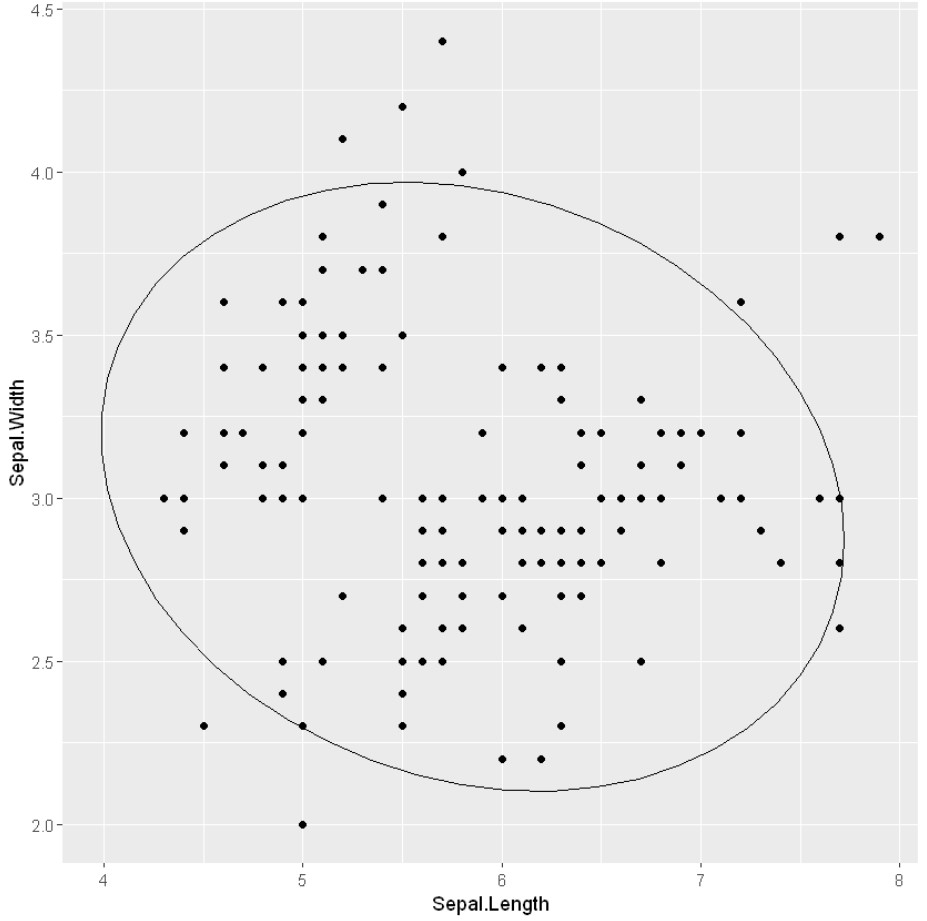My Personal Notes arrow_drop_up
Recommended Articles
Page :